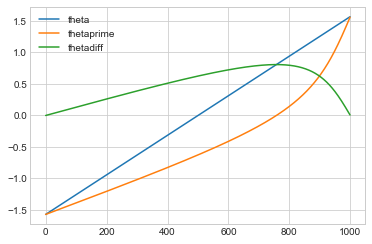# Venus¶

OK. Venus is at its maximum angular separation from the Sun when Earth-Venus-Sun form a right triangle—which is when the Earth-Venus line-of-sight is tangent to the orbit of Venus...

In :
%matplotlib inline

import matplotlib.pyplot as plt
plt.style.use('seaborn-whitegrid')

import numpy as np
import pandas as pd

In :
theta = []
thetaprime = []
thetadiff = []

b = 0.723
pi = 3.141592653

for i in range(-500,500):
thetai = pi*i/1000
y = np.sin(thetai)
x = np.cos(thetai)
yprime = y - b
xprime = x
thetaprimei = np.arctan(yprime/xprime)
thetadiffi = thetai - thetaprimei
theta = theta + [thetai]
thetaprime = thetaprime + [thetaprimei]
thetadiff = thetadiff + [thetadiffi]

data = [('theta', theta),('thetaprime', thetaprime), ('thetadiff', thetadiff)]
venus = pd.DataFrame.from_items(data)
venus.plot()

/anaconda3/lib/python3.6/site-packages/ipykernel_launcher.py:21: FutureWarning: from_items is deprecated. Please use DataFrame.from_dict(dict(items), ...) instead. DataFrame.from_dict(OrderedDict(items)) may be used to preserve the key order.

Out:
<matplotlib.axes._subplots.AxesSubplot at 0x11a122940>## Venus¶